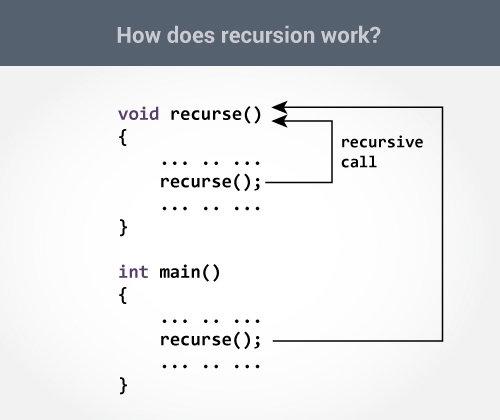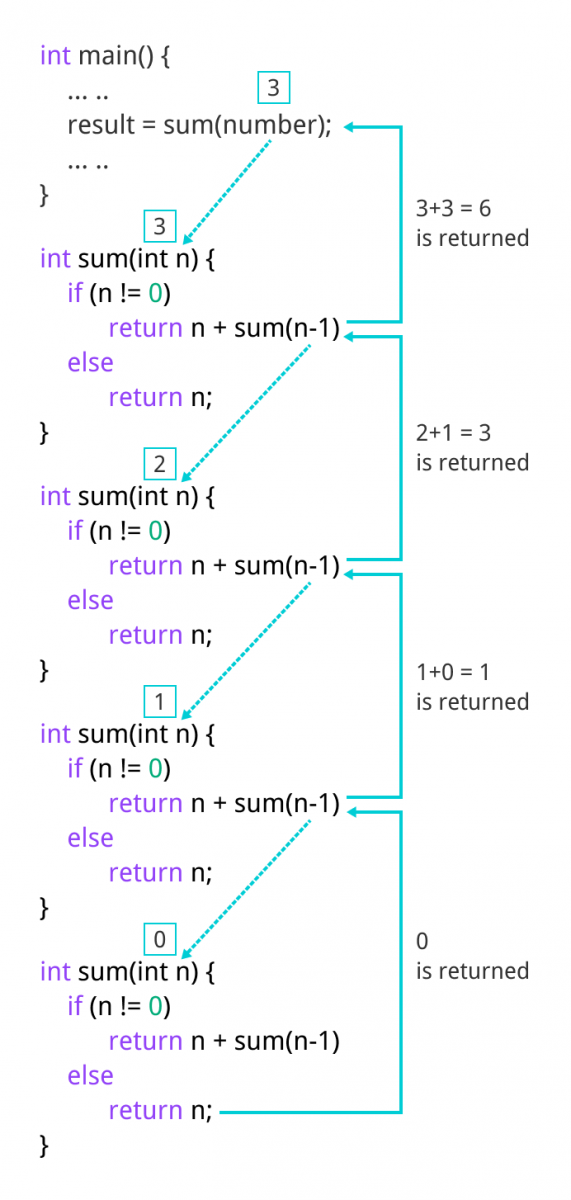C Recursion

# C Recursion

#### In this tutorial, you will learn to write recursive functions in C programming with the help of an example.

A function that calls itself is known as a recursive function. And, this technique is known as recursion.

### How recursion works?

```void recurse()
{
... .. ...
recurse();
... .. ...
}

int main()
{
... .. ...
recurse();
... .. ...
}```The recursion continues until some condition is met to prevent it.

To prevent infinite recursion, if...else statement (or similar approach) can be used where one branch makes the recursive call, and other doesn't.

### Example: Sum of Natural Numbers Using Recursion

``````#include <stdio.h>
int sum(int n);

int main() {
int number, result;

printf("Enter a positive integer: ");
scanf("%d", &number);

result = sum(number);

printf("sum = %d", result);
return 0;
}

int sum(int n) {
if (n != 0)
// sum() function calls itself
return n + sum(n-1);
else
return n;
}
``````

Output

```Enter a positive integer:3
sum = 6```

Initially, the `sum()` is called from the `main()` function with number passed as an argument.

Suppose, the value of n inside `sum()` is 3 initially. During the next function call, 2 is passed to the `sum()` function. This process continues until n is equal to 0.

When n is equal to 0, the `if` condition fails and the `else` part is executed returning the sum of integers ultimately to the `main()` function.